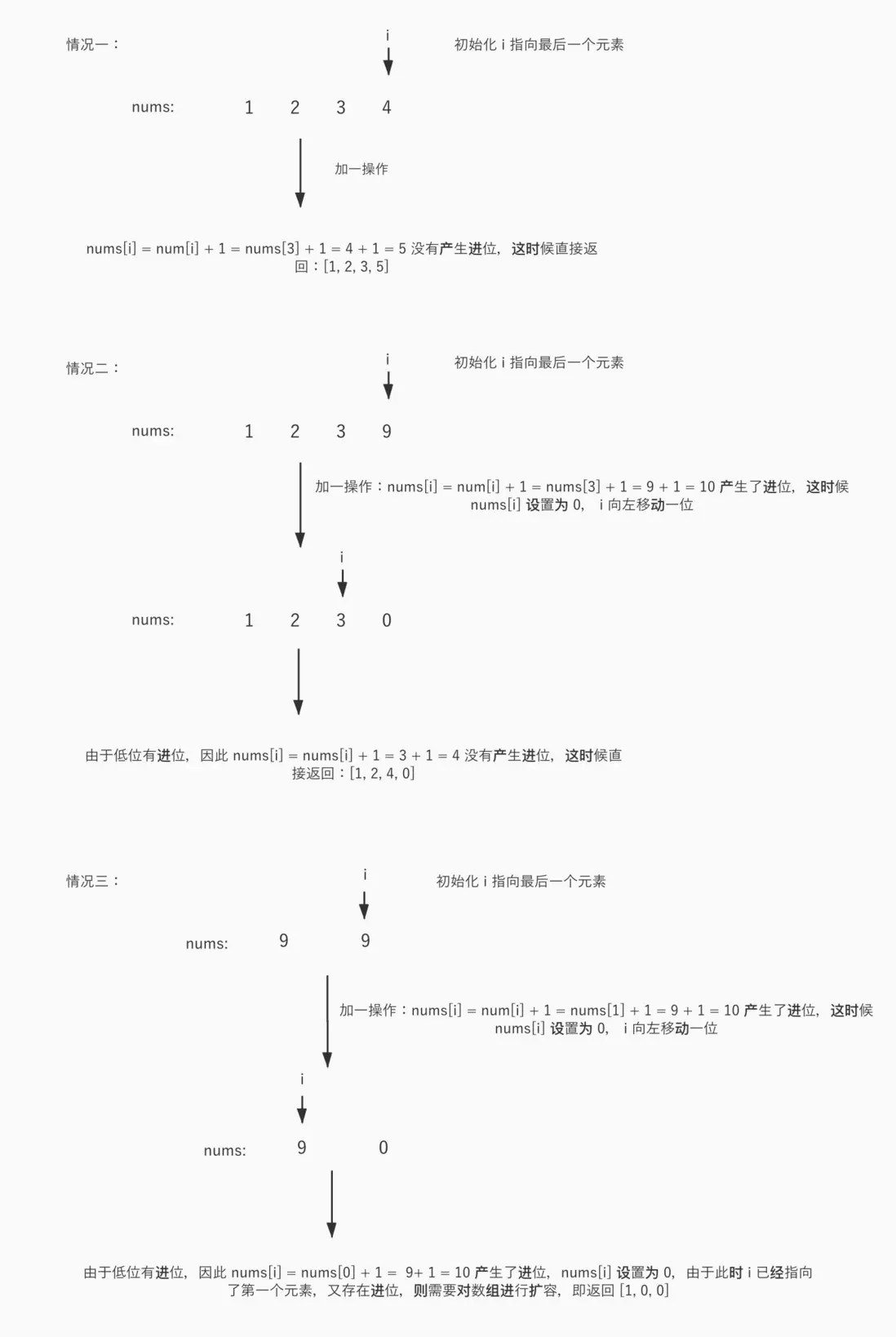# Let's chew the algorithm together-plus one

Posted May 25, 20203 min read

## Plus one(Plus-One)

Question stem:

Given a non-negative integer represented by a non-empty array of integers, add one to the number.
The highest digit is stored in the first digit of the array, and each element in the array stores only a single digit.
You can assume that this integer will not start with zero except for the integer 0.
Example 1:
Input:\ [1,2,3 ]
Output:\ [1,2,4 ]
Explanation:The input array represents the number 123.
Example 2:
Input:\ [4,3,2,1 ]
Output:\ [4,3,2,2 ]
Explanation:The input array represents the number 4321.
Source:Force Buckle

The problem is relatively simple, and the idea of solving the problem is almost the same as Let's nibble the algorithm-add two numbers .

## Problem-solving ideas

According to the intent of the problem, the elements of the array can be regarded as a positive integer with the first element as the highest bit, which is recorded as number, and then add one to the number. Pay attention to carry(carry) question. Since the title clearly states Each element of the array only stores a single number, it can be concluded that only the element value of 9 is likely to produce a carry, and the carry value is 1, the current element value that produces a carry will be assigned 0 **.

The solution to the problem based on the above analysis naturally came out:the preferred initialization i points to the last element of the array. Then add 1 to the positive integer represented by the array, that is, add one to the last element of the array:nums \ [i ]= nums \ [i ]+ 1, and then judge * Whether * nums \ [i ]produces a carry is actually to determine whether nums \ [i ]is equal to 10, or whether nums \ [i ]%10 is equal to 0 . If no carry is generated, the array is returned directly, otherwise, nums \ [i ]= 0, i moves one bit to the left, nums \ [i ]= nums \ [i ]+ 1(because there are low bits If a carry is generated, add 1), then judge whether nums \ [i ]still produces a carry, and repeat the above judgment process **.

Here is a note:When i points to the highest bit, which is the first element, and the first element is 9, and there is also a carry, you need to expand the array. For example, the nums array is \ [9, 9, 9 ]At this time, after adding one, the nums is \ [1, 0, 0, 0 ]**.

The flow chart is as follows:## Code

#### GO language implementation

``````func plusOne(digits []int) []int {
for i:= len(digits) -1; i> = 0; i-- {
//The first increment 1 operation is specified by the topic, and the subsequent increment 1 operation is the low order carry
digits [i]++

//Less than 10 and 10 phase modulus values   remain unchanged, equal to 10 and 10 phase modulus is 0, the main purpose of this step is the current value is 10 when there is a carry, it needs to be set to 0
digits [i]%= 10

//If it is not equal to 0, it means there is no carry, and return directly
if digits [i]! = 0 {
return digits
}

//Equal to 0 means there is a carry, because digits [i]%= 10 is executed above, the current value has been set to 0 at this time, after the subsequent i--, digits [i]++ is executed because there is a carry 1 Need to add
}

//i is out of bounds, and digits is 0 means there is a carry 1, so the array needs to be expanded
digits = append([]int {1}, digits ...)
return digits
}``````

## to sum up

Make a little progress every day, come on!
Algorithm tutorial project, update one question every day, click a star to support it:
https://github.com/wx-satellite/learning-a...